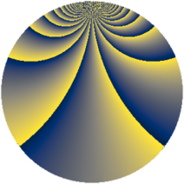# Properties

 Label 552.2.tLevel $552$ Weight $2$ Character orbit 552.t Rep. character $\chi_{552}(19,\cdot)$ Character field $\Q(\zeta_{22})$ Dimension $480$ Newform subspaces $2$ Sturm bound $192$ Trace bound $2$

# Related objects

## Defining parameters

 Level: $$N$$ $$=$$ $$552 = 2^{3} \cdot 3 \cdot 23$$ Weight: $$k$$ $$=$$ $$2$$ Character orbit: $$[\chi]$$ $$=$$ 552.t (of order $$22$$ and degree $$10$$) Character conductor: $$\operatorname{cond}(\chi)$$ $$=$$ $$184$$ Character field: $$\Q(\zeta_{22})$$ Newform subspaces: $$2$$ Sturm bound: $$192$$ Trace bound: $$2$$ Distinguishing $$T_p$$: $$5$$

## Dimensions

The following table gives the dimensions of various subspaces of $$M_{2}(552, [\chi])$$.

Total New Old
Modular forms 1000 480 520
Cusp forms 920 480 440
Eisenstein series 80 0 80

## Trace form

 $$480q + 4q^{2} - 8q^{4} + 4q^{6} + 4q^{8} - 48q^{9} + O(q^{10})$$ $$480q + 4q^{2} - 8q^{4} + 4q^{6} + 4q^{8} - 48q^{9} - 8q^{16} + 4q^{18} + 4q^{24} - 48q^{25} + 4q^{32} - 22q^{34} + 14q^{36} - 110q^{38} + 22q^{40} - 110q^{42} + 154q^{44} - 48q^{46} + 88q^{48} - 48q^{49} - 70q^{50} + 110q^{52} - 18q^{54} + 110q^{56} - 22q^{58} + 22q^{60} + 80q^{62} - 8q^{64} + 4q^{72} - 22q^{74} - 110q^{76} - 198q^{80} - 48q^{81} - 52q^{82} - 220q^{86} - 176q^{88} - 186q^{92} - 190q^{94} + 4q^{96} - 156q^{98} + O(q^{100})$$

## Decomposition of $$S_{2}^{\mathrm{new}}(552, [\chi])$$ into newform subspaces

Label Dim. $$A$$ Field CM Traces $q$-expansion
$$a_2$$ $$a_3$$ $$a_5$$ $$a_7$$
552.2.t.a $$240$$ $$4.408$$ None $$0$$ $$24$$ $$0$$ $$0$$
552.2.t.b $$240$$ $$4.408$$ None $$4$$ $$-24$$ $$0$$ $$0$$

## Decomposition of $$S_{2}^{\mathrm{old}}(552, [\chi])$$ into lower level spaces

$$S_{2}^{\mathrm{old}}(552, [\chi]) \cong$$ $$S_{2}^{\mathrm{new}}(184, [\chi])$$$$^{\oplus 2}$$